Published in Underground Space, Vol. 3 (4), pp. 310-316, 2018
doi: 10.1016/j.undsp.2018.09.002

## Abstract

We present recent developments in the coupling of the finite element method (FEM) and the discrete element method (DEM) for analysis of rock blasting operations in tunnels. The coupled FEM-DEM technique has proven to be an efficient procedure for predicting the multi-fracture of rock induced by the loads generated in blasting situations.

The coupled FEM-DEM procedure is applied in the tunnel construction, as well as to gas pressure blasting pyrotechnics situations to break rock for excavation of the tunnel front. In the latter case the effect of gas explosion is modelled by solving with the FEM the equations of gas dynamics in the analysis domain. The effect of the gas forces in the underlying rock mass is modelled via an embedded fluid-structure interaction method.

The efficiency of the coupled FEM-DEM technique is demonstrated in several examples of fracture tests and rock blasting problems related to tunnel engineering. The examples presented show that the combination of the DEM with simple 3-noded linear triangular elements (for 2D problems) correctly captures the onset of fractures and their evolution accounting for the penetration of the gas in the failure domain [1,2].

Keywords: Discrete elements, finite elements, fracture mechanics, FEM-DEM, gas dynamics.

## 1 Introduction

In this work we extend the simple FEM-DEM procedure presented in [2,1], for analyzing of blasting operation in tunnel fronts leads to multiple fractures and material disgregation of the rock model.

The computed prediction of fractures in a rock induced by a blast load using the finite element method (FEM) poses numerous challenges due to the difficulty of the FEM for computing the onset and propagation of multiple cracks. The problem complexity increases if the effect of the gas pressure acting within the cracks is taken into account [3,4,5].

The FEM-DEM procedure is based on an initial discretization of the analysis domain by a mesh of standard finite elements (FE). Onset of fracture at a point is governed by a simple damage model. Once a crack is detected at an element side in the FE mesh, discrete elements (DE) are generated at the nodes sharing the side. A DEM approach is used to model the evolution of the crack in the fractured solid [3,5,6,7,8]. This optimizes the use of DE to zones where they can be more effective, which considerably simplifies the contact search process and reduces the overall computational cost. Applications of the FEM-DEM technique can be found in [9,2,1].

In this paper we use two methods to simulate the pressure-time behavior of an explosion: a) The direct application of the well known pulse function on the fracture faces, and b) Solution of the gas dynamic equations within the fracture domain coupled to the solid mechanics solution using an embedded mesh approach. Examples of boths numerical procedures to rock blasting solutions are given.

## 2 Basics of the Implementation

### 2.1 FEM DEM approach

We solve the solid domain using an implicit solution in time of the dynamic equilibrium equations. In essence, the adaptive FEM-DEM procedure starts with a discretization of the analysis domain using a FE mesh only and operates as explained next.

1. At each time instant the stress level is verified at each element. For linear triangles and tetrahedra implies computing the strains and stresses at the middle point of the element sides. This strategy follows the idea of the superconvergent patch recovery procedure  and provides a better stress field than the one obtained at the gauss points .

2. The threshold of failure (fracture) of each element is evaluated. This can be done via procedures based on the point-wise value of the stresses (or the strains), or using an adequate energy norm. In our work the rock has a linear elastic behavior limited by a simple Rankine crack model with a linear damage rule which has been used to define the onset of fracture at the mid-point of the element sides . The element damage corresponds to the largest value of the damage parameter at the different cut planes existing in a triangle. The cut plane damage is evaluated as the mean value of the damage parameter at the edges involved in such cut plane as shown in Figure 1(a), were two element sides are damaged.

3. A collection of DE is introduced within the FE that have exceeded the failure threshold. In our work this occurs when element damage reaches a 95% of the threshold value. At this moment, the continuum region previously occupied by the damaged element is modelled with a collection of DE created at the element nodes and the damaged elements are removed Figure 1(b). The radius of the new DE is defined as the maximum possible to avoid collisions with other DE. The mass of the system remains constant because 1/3 of the element mass goes to each DE created at the element nodes.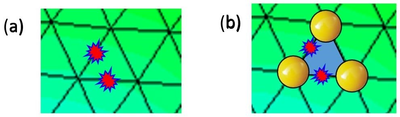Figure 1: (a) Two element sides damaged in a FE mesh. (b) Element removal and DE creation (not in scale) at an element with two sides exceeds the damage threshold.

4. A two-stage time integration scheme is used to solve the contact between the DE and the FE mesh. For the DE an explicit time integration scheme is used to record the impulse between ${\textstyle t}$ and ${\textstyle t+\Delta t}$ [12,13]. The impulse found is used in the FE mesh to evaluate the displacements, velocities and accelerations and also at the DE at the same time period via an implicit time integration scheme. The introduction of additional DE regions on the FE mesh evolves in time accordingly to the evolution of the stress field in the domain. For blast problems the transition of the FE mesh to the DE region occurs quite rapidly, as the fracture zone progresses almost at the blast speed. The adaptive FEM-DEM procedure is still effective in these cases as the time increment for the explicit solution is very small and the delay in introducing DEs leads to considerable savings in computing time span [12,13,2,1].

### 2.2 Simulation of the explosion via a pressure function

In order to characterize the effect of the explosion within the fractured domain two different approaches have been used. The simplest one corresponds to the classical pressure-time function wide used in the literature [14,15,16] which expresses the pressure as an exponential function of time where the parameters used try to reproduce the characteristic print of the more popular explosives.

The amplitude and the duration of the pressure wave can be determined by the internal energy of the explosive and the size and geometry of the borehole. The following general form of a pulse function represents a large range of borehole pressures ,

 $P=P_{0}\left[\exp ^{(-\alpha t)}-\exp ^{(-\beta t)}\right]$
(1)

where ${\textstyle P}$ is the pressure at time ${\textstyle t}$, ${\textstyle P_{0}}$ is the peak pressure, and ${\textstyle \alpha }$ and ${\textstyle \beta }$ are constants. The pressure-time curves for the ratio ${\textstyle \alpha /\beta =2}$ used in this work are depicted in Figure 2.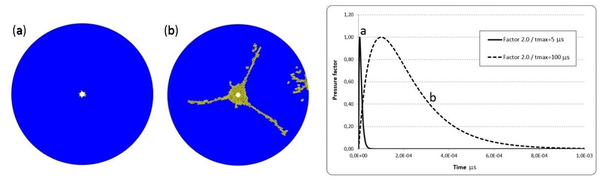Figure 2: Effect of pressure loading rate. (a) $20$ MPa/µs loading rate. (b) $1$ MPa/µs loading rate. The graphs shows the two pressure curves used

### 2.3 Solution of the compressible gas equations

The effect of the (compressible) gas within the fractures is modeled by solving the Euler equations, given by

 ${\boldsymbol {u}}_{,t}+\nabla \cdot {\boldsymbol {F}}=0$
(2)

 ${\boldsymbol {u}}=\left[\rho \;;\;\rho v_{i}\;;\;\rho e\right]^{T}$
(3)

 ${\boldsymbol {F}}_{j}=\left[\rho v_{j}\;;\;\rho v_{i}v_{j}+p\delta _{ij}\;;\;v_{j}(\rho e+p)\right]^{T}$
(4)

Remark 1: Uppercase variables in boldface represents matrices and lowercase variables in boldface corresponds to vectors.

where ${\textstyle {\boldsymbol {u}},{\boldsymbol {F}},\rho ,v_{i},e,p}$ denote, respectively, the vector of unknowns, the flux vector, the density, the velocity , the energy and the pressure. The Galerkin finite element discretization of the Equations 5 and 6, written in edge-based form , yields a discrete set of ordinary differential equations (ODEs) of the form:

 $M^{ij}{\hat {u}}_{,t}^{j}=C^{ij}{\cal {F}}_{ij}$
(5)

here, ${\textstyle {\boldsymbol {M}},{\hat {u}}^{j},C^{ij},{\cal {F}}^{ij}}$ denote the mass-matrix, the vector of nodal unknowns, the edge-coefficients for fluxes and the edge-fluxes respectively. The Galerkin edge-fluxes are replaced by numerically consistent fluxes, typically given by approximate Riemann solvers (van Leer, Roe, HLLC,...). Monotonicity is imposed either by limiting the variables before flux evaluation (van Leer, van Albada, minmod,...), or after flux evaluation via flux-corrected transport (FCT) techniques [18,19,20,21]. The ODEs given by Eq. 5 are integrated in time using explicit Runge-Kutta schemes of higher order (typically 3,4), or via Taylor-Galerkin techniques. The runs shown were conducted with FEM-FCT techniques.

The pressure is obtained from various equations of state. For the gas we have used, either the ideal gas equation of state, given by

 $p=(\gamma {-1)}\rho [e-{1 \over 2}v_{j}v_{j}]$
(6)

or a table look-up ${\textstyle p=p(\rho ,e_{i})}$, which may also be interpreted as a non-constant ${\textstyle \gamma }$.

The high explosive material (HEM) is modeled with the Jones-Wilkins-Lee (JWL) equation of state [22,23], given by:

 $p=A\left(1-{\omega \over {R_{1}v}}\right)e^{-R_{1}v}+B\left(1-{\omega \over {R_{2}v}}\right)e^{-R_{2}v}+\omega \rho e_{i}$
(7)

where ${\textstyle v}$ denotes the relative volume of the gas:

 $v={V \over V_{0}}={\rho _{0} \over \rho }$
(8)

note that the equivalent ideal gas value of ${\textstyle \gamma }$ is given by ${\textstyle \gamma =1+\omega }$. However, for many materials ${\textstyle \omega }$ is quite far from ${\textstyle \gamma _{air}=1.4}$. Therefore, a combination of EOS is employed once the density of the HEM falls below a threshold or switch value.

Afterburning is modelled by adding energy via a burn coefficient ${\textstyle \lambda }$ that is obtained from

 $\lambda _{,t}=ap^{1 \over 6}{\sqrt {1-\lambda }}$
(9)

after updating ${\textstyle \lambda }$, the energy added is given by:

 $\left.\rho e\right|^{n+1}=\left.\rho e\right|^{n}+\rho Q(\lambda ^{n+1}-\lambda ^{n})$
(10)

where ${\textstyle Q}$ is the afterburn energy.

## 3 Coupled gas-solid solution via embedded mesh technique

At each timestep, the computational solid dynamics (FEM-DEM) code transfers to the compressible gas flow solver the `wetted faces' of the (cracked) domain. The flow solver detects the edges crossed by the wetted faces and applies proper boundary conditions. A number of embedded grids for solvers of this kind have been developed over the last two decades; see [24,25,26,4,27,28,29,30,31] for a detailed description.

## 4 Examples

Many examples in 2D and 3D have been performed in order to verify the accuracy and robustness of the FEM-DEM formulation for analysis of multifractures in solids, all of them with excellent results [2,1]. Herein some examples related to rock blasting problems are present in order to show the usefulness of the FEM-DEM procedure in this field.

It is well known that the mechanical properties of rock materials are affected by the strain rate. This effect has a big influence in the rock fracture pattern. Higher strain rates produce a crushed zone and short fractures, while lower strain rates produce a small crushed zone and fewer and longer radial fractures [16,15].

To verify these observations of the strain rate effect, two different waveforms of the blast loading have been numerically simulated with the FEM-DEM technique and compared with literature results . Figure 2 shows the pressure–time record used for the numerical simulation. The 2D model consists of a borehole (${\textstyle \phi =0.005}$m) in a circular rock mass (${\textstyle \phi =0.140}$m) modeled with ${\textstyle 35,046}$ elements. The material properties are E= ${\textstyle 21.0}$ GPa, ${\textstyle \nu =0.22}$, ${\textstyle \gamma =25,000}$N/m${\textstyle ^{3}}$, thickness = ${\textstyle 0.1}$ m, ${\textstyle \sigma _{t}=5.23}$ MPa and ${\textstyle G=22}$ J/m${\textstyle ^{2}}$. The pressure rising time ${\textstyle t_{0}}$ varies between ${\textstyle 5}$ and ${\textstyle 100}$ µs. The peak pressure is kept constant to ${\textstyle 100}$ MPa. while the loading rates are ${\textstyle 20}$ and ${\textstyle 1.0}$ MPa/µs, respectively.

The simulated fracture patterns with different loading rates are compared in Figure 2. When the loading rate of the pressure is high (${\textstyle 20}$ MPa/µs), only a small crushed zone is created Figure 2(a). The blast energy is primarily dissipated in creating the crushed zone. When the loading rate decreases to ${\textstyle 1.0}$ MPa/µs, a central crushed zone and longer radial fractures appear, as shown in Figure 2(b). The results agree with those reported in [15,16,32].

### 4.2 Open air quarry analysist

The geometry analyzed corresponds to a ${\textstyle 4.0\times 6.25}$ m 2D domain constrained at all its edges except the lower one. The cutting line was set at ${\textstyle 1.0}$ m from the free side. Different spacings of the boreholes (${\textstyle \phi =0.175}$ m) were analyzed (${\textstyle 0.25}$, ${\textstyle 0.50}$, ${\textstyle 0.75}$ and ${\textstyle 1.0}$ m). The load pressure is defined as in curve (b) of Figure 2. The material properties are ${\textstyle E=10}$ GPa, ${\textstyle \nu =0.2}$, ${\textstyle \gamma =78,000}$ N/m${\textstyle ^{3}}$, thick = ${\textstyle 1.0}$ m, ${\textstyle \sigma _{t}=0.10}$ MPa and ${\textstyle G=100}$ J/m${\textstyle ^{2}}$.

The results obtained show a clear correlation (${\textstyle >90\%}$) of the width of the damaged zone respect the borehole spacing, which goes from ${\textstyle 0.2}$m for the ${\textstyle 0.25}$ m spacing up to ${\textstyle 0.6}$ m for ${\textstyle 1.0}$ m spacing, as shown in Figure 3. This results are the expected ones and confirm the good prediction capabilities of the FEM-DEM simulation.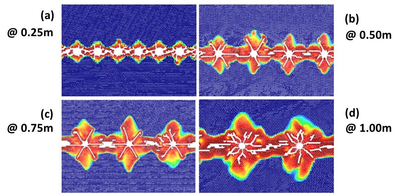Figure 3: Open air quarry analysis. Damage zone and cracks around the boreholes. Four different spacings (in meters) are shown.

### 4.3 “Aguas Teñidas” tunnel front

“Aguas Teñidas” is a mine located in the province of Huelva, Spain. It is a conformant sulfide deposit of the Iberian Pyrite Belt. This mine produces copper and polymetallic. The exploitation is carried out using mainly conventional drilling equipment for blasting.

A front blasting sequence is analyzed with the pressure rising time of ${\textstyle 100}$ µs. and a peak pressure of ${\textstyle 100}$ MPa. The material properties correspond to ${\textstyle E=10}$ GPa, ${\textstyle \nu =0.2}$, ${\textstyle \sigma _{t}=0.10}$ MPa and ${\textstyle G=100}$ J/m${\textstyle ^{2}}$.

The tunnel front has ${\textstyle 57}$ boreholes classified in ${\textstyle 8}$ blasting groups as shown in Figure 4(a). The blasting delay is ${\textstyle 15}$ µs between each group. No initial pressure is applied at the rock mass; This means that the tunnel is assumed to be at a very low depth. On Figure 4(b) the damage at the final time of analysis is presented. It is remarkable the sub-excavation at the base of the tunnel which matches the real problem. The horizontal damage zone is due to the zero initial pressure in the rock mass.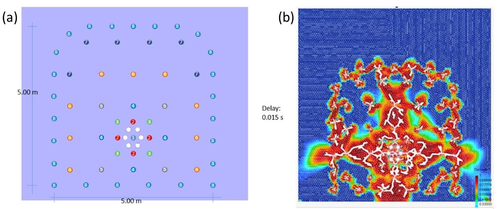Figure 4: Aguas Teñidas tunnel front. (a) Blasting sequence. (b) Crack and damage pattern.

### 4.4 Coupled blast-crack problem

A simple example is shown for the coupled solution between the cracking in a square solid rock mass and the CFD analysis in which the explosive state equations are solved coupled to the FEM-DEM solution. The example corresponds to a 2D granite rock sample of ${\textstyle 0.2\times 0.2}$ m and a borehole of ${\textstyle \phi =0.02}$m. The explosive used corresponds to a C4 kind. Besides the simplicity of the example, it proves the usefulness of the coupling blast-cracking scheme proposed.

In Figure 5 the cracks and damage zone for two different times (${\textstyle 41}$ µs and ${\textstyle 82}$ µs) are presented. The cracked domain is passed to the CFD solver each time step in order to compute the pressure evolution in the gas zone which value is returned to the FEM-DEM solver for the solid mechanical analysis.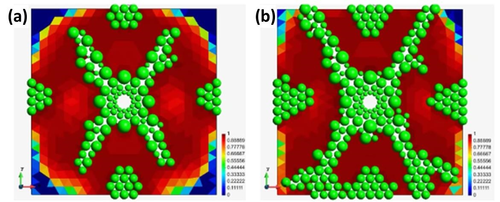Figure 5: Damage zone and cracks on the specimen at (a) $41$ µs and (b) $82$ µs.

Figures 6 and 7 shown the CFD results for the same times. Figures (a) display the fluid velocity and Figures (b) the pressure evolution in the gas zone within the cracks. We highlight the shockwaves produced by the explosion within the gas domain.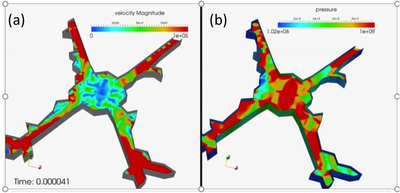Figure 6: (a) Velocity and (b) pressure distribution in the gas domain at $41$ µs.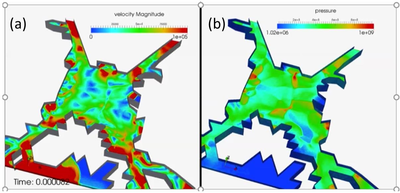Figure 7: (a) Velocity and (b) pressure distribution in the gas domain at $82$ µs.

## 5 Conclusions

A simple FEM-DEM methodology has been presented for reproducing the cracking pattern in rock blasting problems. The good results obtained indicate that the FEM-DEM technique is an efficient and promising procedure for solving rock excavation and blasting situations in tunnels.

In addition to the standard pressure-time functions, the effect of the gas forces whitin the fractures in the underlying rock mass has been modelled with an embedded fluid-structure interaction method. This couppled procedure accurately predicts the progressive multi-fracture of the rock accounting for the penetration of the gas in the fractured domain modelled with the FEM-DEM procedure described

## 6 Acknowledgements

The authors acknowledge the financial support of the Tuñel project funded by the Spanish Ministry of Science and Innovation. (2015-2019) via the subprogram CIEN (IDI-20150705) and also the support of the EZUANA project (BIA2016-78544-R), funded by the Spanish Ministry of Education and Science of Spain.

## 7 Conflict of interest

On behalf of all authors, the corresponding author states that there is no conflict of interest.

### Document informationPublished on 11/02/19
Submitted on 11/02/19

DOI: 10.1016/j.undsp.2018.09.002

### Document Score0

Times cited: 1
Views 7
Recommendations 0